# How to compare two columns for (highlighting) missing values in Excel?

Often we have multiple sheets of dataset having similar values but not aware whether any value is missing or not. Manually checking each value will be a tedious activity. So for this we can use the below mentioned formula which will identify the missing values in no time. Let’s see how to implement this formula.

## Compare Two Columns for Missing Values with Formulas and Highlighting the Same

Step 1  We have taken the below sample data in different sheets for comparison having some common as well as different values.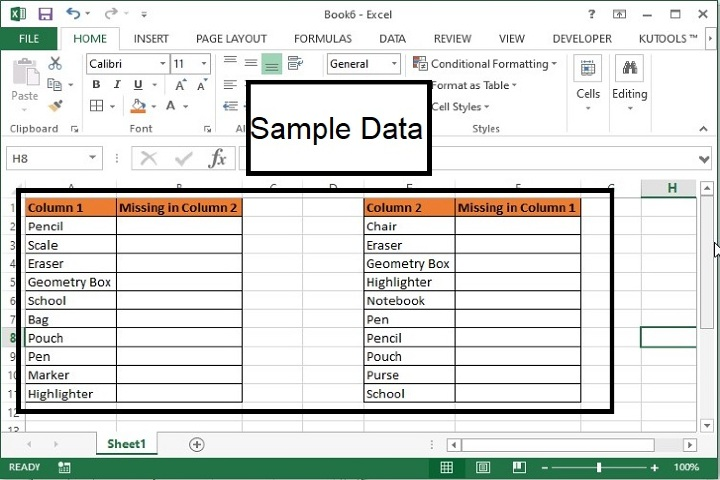Here, we have taken some data in two separate columns and against each column, the missing items in another columns will be highlighted.

Step 2  Now, enter the following formula in the column named as, ‘Missing in Column 2’.

=IF(ISERROR(VLOOKUP(A2,$E$2:$E$11,1,FALSE)),A2,"")


Note −

• In above formula, A2 is the value in Column 1, $E$2:$E$11 is the range whose missing values you are looking for.

• This formula is not case sensitivity.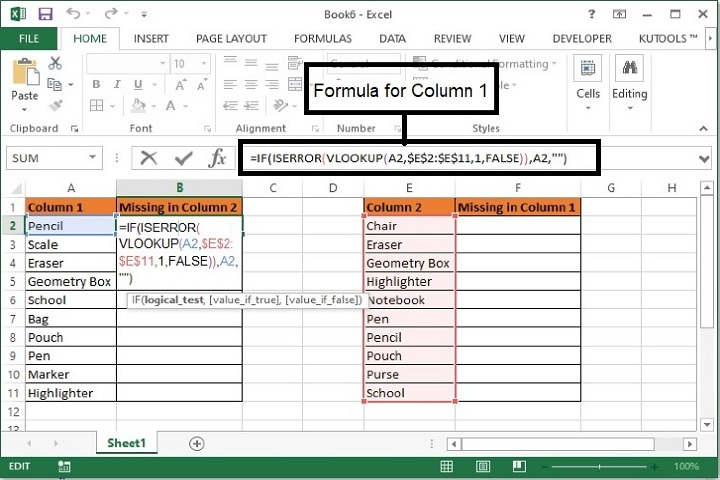Step 3  Now drag the formula till the last row and the missing value in Column2 will be printed as shown in the below screenshot.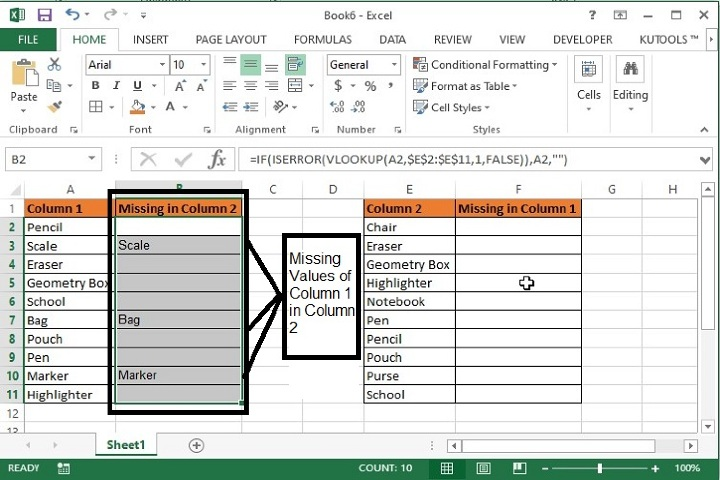Step 4 − Similarly, enter the following formula in the column named as, “Missing in Column 1”.

=IF(ISERROR(VLOOKUP(E2,$A$2:$A$11,1,FALSE)),E2,"")


Note −

• In above formula, E2 is the value in Column 2, $A$2:$A$11 is the range whose missing values you are looking for.

• This formula is not case sensitivity.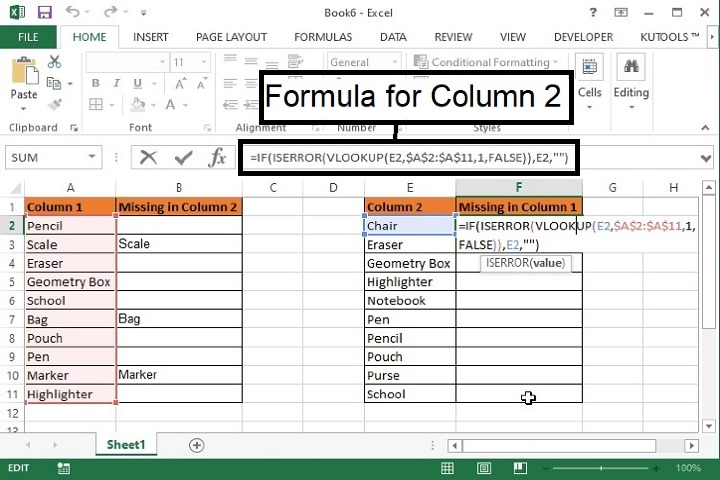Step 5  The compared data will be displayed as shown below.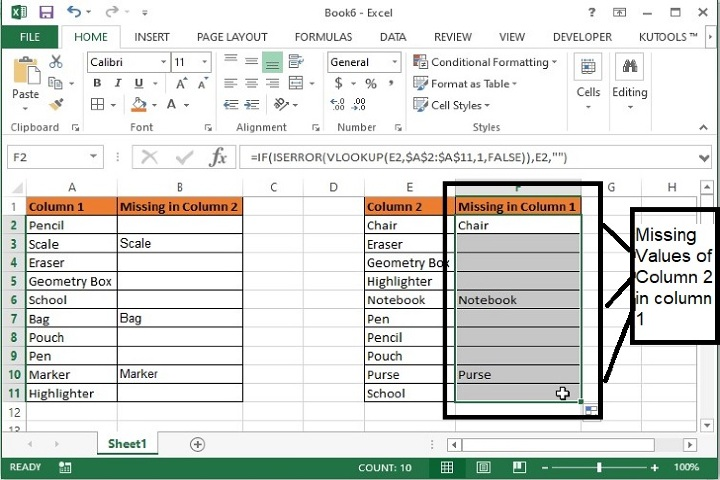Step 6  Now to highlight the values select the missing values and fill the color. Select the missing cells and go to Home / Font / Fill Color and choose the desired color to fill in.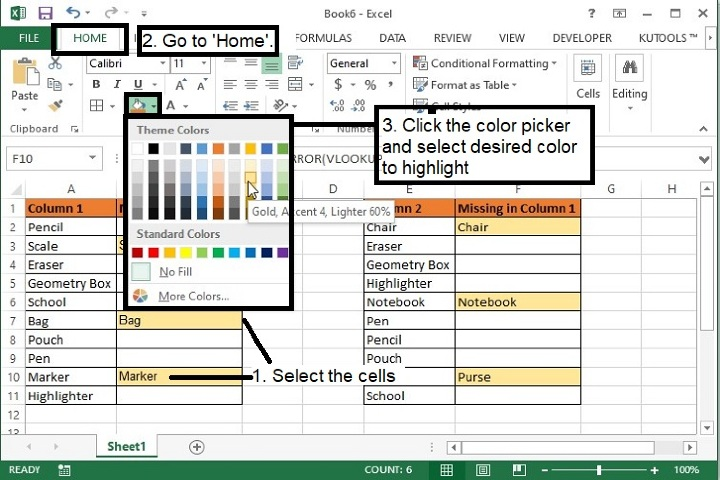Step 7  So the final output will be displayed as shown below.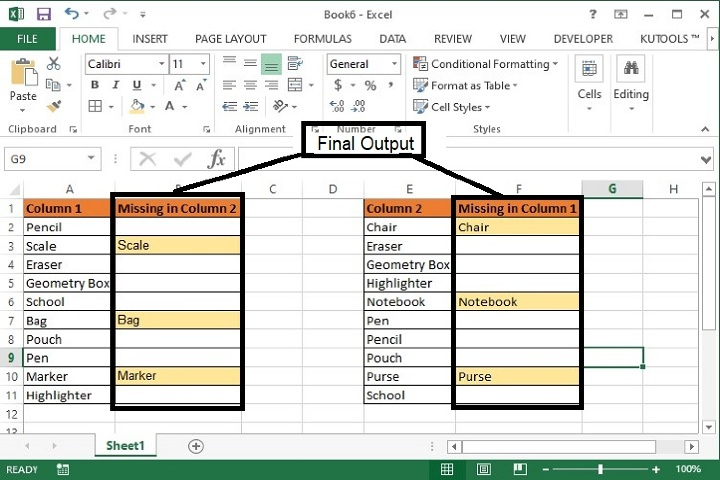## Conclusion

Hence in this way you can identify the missing values in multiple column datasets and highlight the same. The same can be achieved using Kutools extension as well but as that is a subscription based feature so I will suggest you to go with the excel feature itself. Keep learning and keep exploring excel.

Updated on: 02-May-2023

205 Views